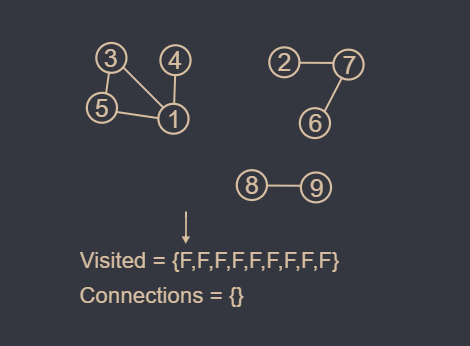20 July 2021

Statement

( The original statement can be found here )
Byteland has n cities, and m roads between them. The goal is to construct new roads so that there is a route between any two cities. Your task is to find out the minimum number of roads required, and also determine which roads should be built.

Input
The first input line has two integers n and m: the number of cities and roads. The cities are numbered 1,2,…,n. After that, there are m lines describing the roads. Each line has two integers a and b: there is a road between those cities. A road always connects two different cities, and there is at most one road between any two cities.

Output
First print an integer k: the number of required roads. Then, print k lines that describe the new roads. You can print any valid solution.

Constraints
1 ≤ n ≤ 10^5
1 ≤ m ≤ 2 x 10^5
1 ≤ a,b ≤ 10^5
Time limit : 1.00s
Memory limit : 512MB

Example

Input :
4 2
1 2
3 4

Output :
1
2 3

Solution

The first question one might ask is when are two cities unreachable from each other ? If we separate the cities by groups where each group is only composed of cities that are reachable from each other we will get the following representation.(Hint : The image above contains 2 groups and only requires one connection to make every city reachable from each other).

As the image suggests, cities belonging to different groups are not reachable, otherwise only one group would exist. If we consider this network of cities as a graph, it becomes a classical problem. The groups become connected components and all we are trying to do is figure out how many there are and which nodes we choose to connect.

To solve this problem, we could use an algorithm like depth-first search to recursively traverse all the nodes in each component and mark them as visited. By doing so, we should be able to count the number of components. Knowing the number of components is crucial since the idea behind the solution is to connect the components minimizing the extra number of connections needed.

The next step is to find which nodes we should connect. One way of finding out is to choose the nodes that weren't yet visited in the iteration process. As unvisited nodes weren't explored by the depth-first search algorithm, we can assume it belongs to a new component.

After choosing the list of nodes to connect we will make a connection for every pair of adjacent nodes in the list. So if the list was {1,5,3} we would connect {1,5} and {5,3}. By doing so we ensure that each component is connected to every other component with the minimum amount of connections. Here is a visual representation of the algorithm.```#include <bits/stdc++.h>
using namespace std;

#define MAXN 202020

int n, m;
vector<bool>visited(MAXN, false);
vector<int>bridges;

void DFS (int node) {
visited[node] = true;
for (auto child : adj[node]) {
if (visited[child] == false) {
DFS(child);
}
}
}

int main() {
cin >> n >> m;
for (int i = 0 ; i < m; i++) {
int node1, node2;
cin >> node1 >> node2;
}
for (int i = 1; i <= n; i++) {
if (visited[i] == false) {
bridges.push_back(i);
DFS(i);
}
}
cout << bridges.size() - 1 << endl;
for (int i = 0; i < bridges.size() - 1; i++) {
cout << bridges[i] << " " << bridges[i + 1] << endl;
}
return 0;
}
```

The time and space complexity are both O(N), since any node is visited only once and we use linear memory based on the number of nodes.